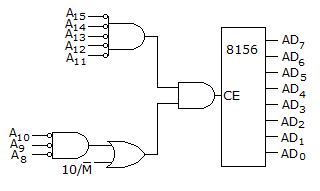# Electronics and Communication Engineering - Digital Electronics

26.

If the switch is thrown to the START position, Q and Y will be equal to

 A. Q = 0, Y = 0 B. Q= 1, Y= 1 C. Q = 1, Y = CLK D. Q = 0, Y = CLK

Explanation:

No answer description available for this question. Let us discuss.

27.

 A. widely used in analyzing and programming microprocessors B. an obsolete system no more in use C. used in calculators only D. none of the above

Explanation:

No answer description available for this question. Let us discuss.

28.

Mark the false statement:

 A. since wave-shape of a digital signal is unimportant, linear amplifiers are not required B. the precise amplitude of a digital signal is also unimportant provided two voltage levels (0 and 1) are quite different C. the leading and trailing edges of a digital signal need not be sharp D. actual digital waves have rise and fall times of the order of a few nano seconds

Explanation:

No answer description available for this question. Let us discuss.

29.

The stack is a specialized temporary __________ access memory during __________ and __________ instructions.
The 8156 of a figure has RAM locations from 2000 H to 20 FFH.A. random, store, load B. random, push, load C. sequential, store, pop D. sequential, push, pop

Explanation:

No answer description available for this question. Let us discuss.

30.

The 54/74164 chip is an 8-bit serial-input-parallel-output shift register. The clock is 1 MHz. The time needed to shift a 8-bit binary number into the chip is

 A. 1 μs B. 2 μs C. 8 μs D. 16 μs